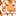# QlikView App Dev

Discussion Board for collaboration related to QlikView App Development.

Announcements
Action-Packed Learning Awaits! QlikWorld 2023. April 17 - 20 in Las Vegas: REGISTER NOW
cancel
Showing results for
Did you mean:Creator

## minYear to Maxyear (YTD)

hi,

I'm new to Qlikview and am trying to get something I thought was relatively simple but seems bit difficult.please help me to get this.

i have year field(FY)-2016,2017,2018,2019

Variables : vMinyear and vMaxyear

have to calculate Revenue

suppose if i select 2016 output should be 2016 to 2019

if 2017: 2017 ,2018, 2019

2018: 2018 to 2019

i have tried like below

sum({<FY={">=\$(vMinyear)<=\$(vMaxyear)"}>}Revenue) but it showing selected year only.

same for  Quarter

Please let me know how to achieve this via set analysis.

Chandra

24 RepliesCreator
Author

Variable is done in variable  overview as same as we discussed.

but still it is showing single year value.

=sum({<FY={">=\$(vMinyear)<=\$(vMaxyear)"}>}Revenue)

Thanks,

ChandraMaster II

I have attached a sample.

Learning never stops.Creator
Author

Hi,

Thanks Pardosh .

Its working Fine .Same as like Year i want to create for Quarter.

Thanks you very much.

ChandraCreator
Author

Hi Pardosh,

help me for QTD also

Thanks ChandraMaster II

How does your quarter data looks like ?

Learning never stops.Creator
Author

Quarter field is Quarter

Q1,Q2,Q3 and Q4 are the values

above expression :

=sum({<FY={">=\$(vMinyear)<=\$(vMaxyear)"}>}Revenue) is working fine

but the expression has to give like below

=If(Group='revenue',=sum({<FY={">=\$(vMinyear)<=\$(vMaxyear)"}>}Revenue),

if(Group='expense',sum({<FY={">=\$(vMinyear)<=\$(vMaxyear)"}>}Expense))

Above xpression is showing the value for the selected year.

Thanks

ChandraMaster II
```=If(Group='revenue',sum({<FY={">=\$(vMinyear)<=\$(vMaxyear)"}>}Revenue),

if(Group='expense',sum({<FY={">=\$(vMinyear)<=\$(vMaxyear)"}>}Expense)
))```
Learning never stops.Creator
Author

Still, it's Showing the selected year in the report.

PFA below.

Expression Is :

=If(Group='REVENUE_Flash' ,sum({<FY={">=\$(vMinyear)<=\$(vMaxyear)"},Flag={1}>}Revenue),

if(Group='EXPENSE_GL',sum({<FY={">=\$(vMinyear)<=\$(vMaxyear)"}>}MORvalue)
))

Thanks,

ChandraMaster II
```=If(Group='REVENUE_Flash' ,sum({1<FY={">=\$(vMinyear)<=\$(vMaxyear)"},Flag={1}>}Revenue),

if(Group='EXPENSE_GL',sum({1<FY={">=\$(vMinyear)<=\$(vMaxyear)"}>}MORvalue)
))```
Learning never stops.Creator
Author